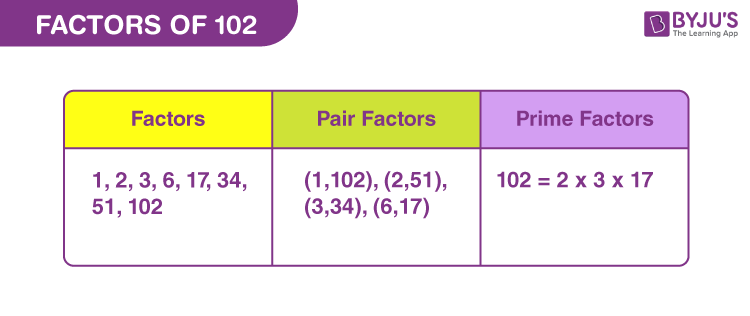# Factors of 102

In Mathematics, factors of 102 are the natural numbers that uniformly divide the original number. It means 102 is divided into equal parts. These factors are also called the divisors of 102. 102 is an even composite number, thus it will have more than two factors.

102 is the first three-digit even number. If the multiplication of two numbers results in 102, then they are the factors of 102. 102 can be represented as pair factor form and prime factor form. Let us find the factors here in this article.## How to Find the Factors of 102?

To find the factors of 102, we have to divide 102 by the numbers that can evenly divide 102.

• 102 ÷ 1 = 102
• 102 ÷ 2 = 51
• 102 ÷ 3 = 34
• 102 ÷ 6 = 17
• 102 ÷ 17 = 6
• 102 ÷ 34 = 3
• 102 ÷ 51 = 2
• 102 ÷ 102 = 1

Therefore, there are a total of eight factors of 102: 1, 2, 3, 6, 17, 34, 51 and 102.

## Pair Factors of 102

Pair factors when multiplied together, results in the original number. Hence, pair factors of 102 are represented as:

• 1 × 102 = 102
• 2 × 51 = 102
• 3 × 34 = 102
• 6 × 17 = 102

Therefore, the pair factors of 102 are (1, 102), (2, 51), (3, 34) and (6,17).

The negative pair factors can be written as the product of two negative numbers that result in 102.

• -1 × -102 = 102
• -2 × -51 = 102
• -3 × -34 = 102
• -6 × -17 = 102

Therefore, the negative pair factors are (-1, -102), (-2, -51), (-3, -34) and (-6,-17).

## Prime Factorisation of 102

The prime factors of 102 can be determined by the prime factorisation method. We need to divide 102 by the prime numbers unless we get the quotient equal to 1.

 102 ÷ 2 = 51 51 ÷ 3 = 17 17 ÷ 17 = 1

Therefore, the prime factors of 102 are 2, 3 and 17.

 Prime factorisation of 102 = 2 x 3 x 17 Exponential form = 21 x 31 x 171

## Solved Examples

Q.1: What should be multiplied by 17 to get 102?

Solution: Suppose, n is the number to be multiplied by 32 to get 102.

So, n × 17 = 102

n = 102/17

n = 6

Therefore, 6 should be multiplied by 17 to get 102 as the result.

Q.2: Find the sum of factors of 102. Also, find the factors of the resulting sum.

Solution: The factors of number 102 are 1, 2, 3, 6, 17, 34, 51 and 102.

Sum of all the factors = 1 + 2 + 3 + 6 + 17 + 34 + 51 + 102

Sum = 216

Therefore, 216 is the required sum.

Factors of 216 = 1, 2, 3, 4, 6, 8, 9, 12, 18, 24, 27, 36, 54, 72, 108, 216

Q.2: What is the greatest common factor of 100 and 102?

Solution:

The factors of 100 are 1, 2, 4, 5, 10, 20, 25, 50, 100

The factors of 102 are 1, 2, 3, 6, 17, 34, 51, 102

Therefore, the greatest common factor of 100 and 102 is:

GCF (100, 102) = 2

### Practice Questions

1. What are the divisors of 102?
2. Find the common factors of 102 and 132.
3. What is the GCF of 102 and 136?
4. Find the GCF of 120 and 102.

## Frequently Asked Questions on Factors of 102

### What are the factors of 102?

The factors of 102 are 1, 2, 4, 8, 16, 32, 64 and 102.

### How many ways can you make 102?

102 can be written as product of two numbers, in four ways:
1 x 102
2 x 51
3 x 34
6 x 17

### What is the 5th multiple of 102?

The fifth multiple of 102 is 510.

### What is the prime factor form of 102?

The prime factor form of 102 is 2 x 3 x 17

### Is 102 a perfect cube?

102 is not a perfect cube. The cube of no natural number results in 102.

### Is 102 a composite number or prime number?

102 is a composite number because it has more than two factors.